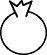EnchantedLearning.com is a user-supported site.
As a bonus, site members have access to a banner-ad-free version of the site, with print-friendly pages.

 You might also like: Divide Fruit into Four Groups Worksheet Printout Divide Fish by Five Worksheet Printout Divide Fruit into Two Groups Worksheet Printout Divide Six by 2, 3, and 6 Worksheet Printout Divide Eight Birds Worksheet Printout Today's featured page: Math

 Go to the AnswersMath Pages EnchantedLearning.com Divide Fruit into Three Groups Divide the fruit into groups to do division. Visual Division PagesDivision Pages

 Divide the bananas into three equal groups.How many bananas are there? How many groups of bananas are there? How many bananas are in each group?
3 ÷ 3 =

 Divide the apples into three equal groups.How many apples are there? How many groups of apples are there? How many apples are in each group?
6 ÷ 3 =

 Divide the blueberries into three equal groups.How many blueberries are there? How many groups of blueberries are there? How many blueberries are in each group?
9 ÷ 3 =

## Enchanted Learning Search

 Search the Enchanted Learning website for: